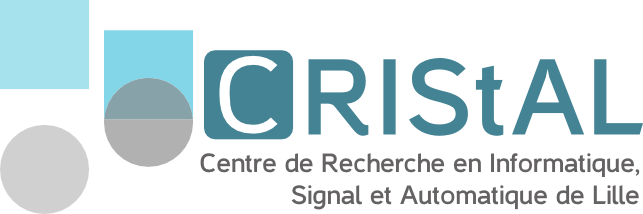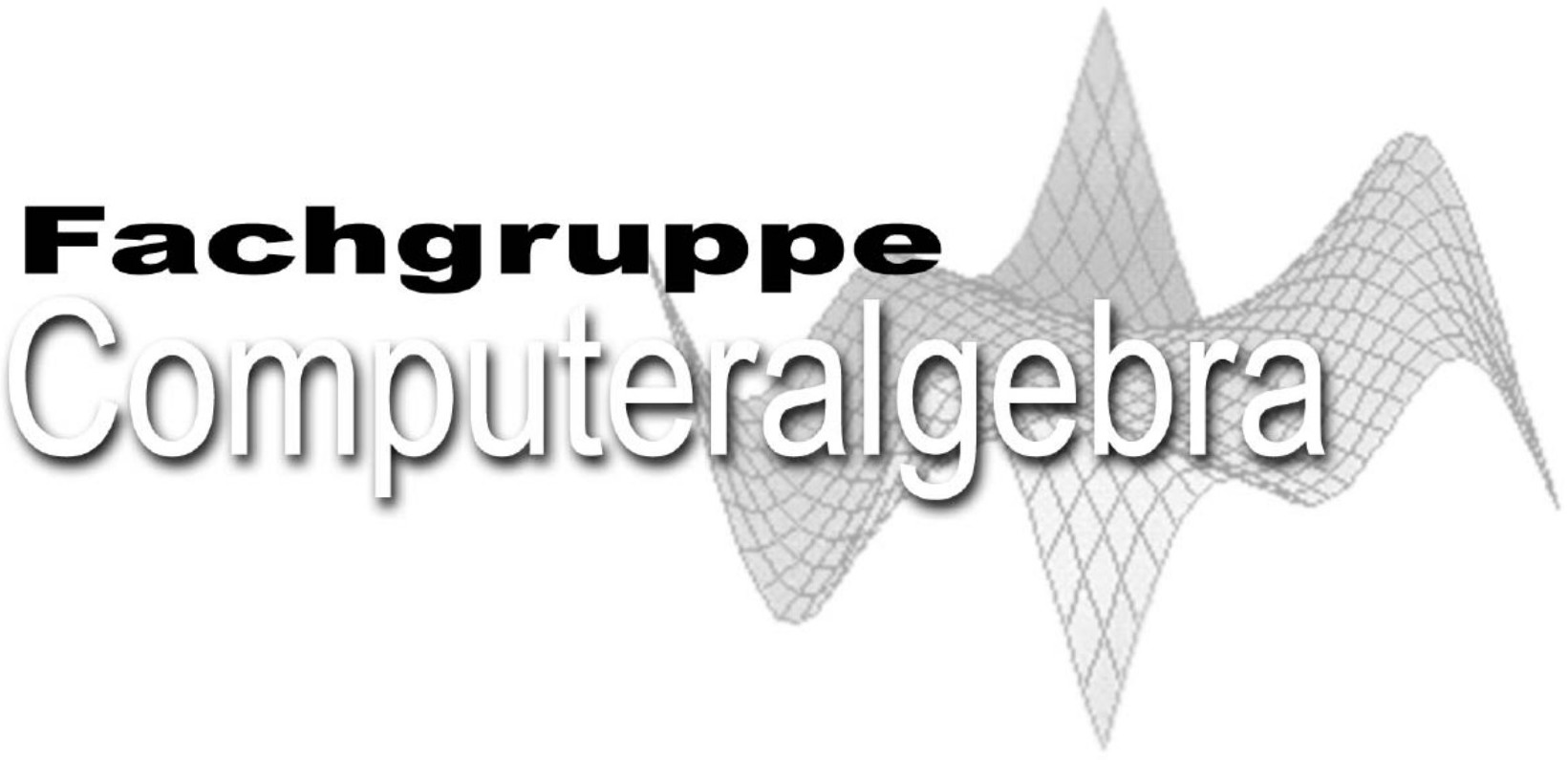# Tutorial Speakers

## Titles and Abstracts

Matías Bender
TU Berlin, Germany### Solving sparse polynomial systems using Gröbner bases and resultants

Abstract:

Solving systems of polynomial equations is a central problem in nonlinear and computational algebra. Since Buchberger's algorithm for computing Gröbner bases in the 60s, there has been a lot of progress in this domain. Moreover, these equations have been employed to model and solve problems from diverse disciplines such as biology, cryptography, and robotics. Currently, we have a good understanding of how to solve generic systems from a theoretical and algorithmic point of view. However, polynomial equations encountered in practice are usually structured, and so many properties and results about generic systems do not apply to them. For this reason, a common trend in the last decades has been to develop mathematical and algorithmic frameworks to exploit specific structures of systems of polynomials.

Arguably, the most common structure is sparsity; that is, the polynomials of the systems only involve a few monomials. Since Bernstein, Khovanskii, and Kushnirenko's work on the expected number of solutions of sparse systems, toric geometry has been the default mathematical framework to employ sparsity. In particular, toric geometry is the crux of the matter behind the extension of classical tools to solve polynomial systems, such as resultant computations, homotopy continuation methods, and most recently, Gröbner bases. In this tutorial, I will review these classical tools, their extensions, and recent progress in exploiting sparsity for solving polynomial systems.

Aydın Buluç
EECS Berkeley, USA### Sparse matrices powering three pillars of science: simulation, data, and learning

Abstract:

In addition to the traditional theory and experimental pillars of science, we are witnessing the emergence of three more recent pillars, which are simulation, data analysis, and machine learning. All three recent pillars of science rely on computing but in different ways. Matrices, and sparse matrices in particular, play an outsized role in all three computing related pillars of science, which will be the topic of my talk.

Solving systems of linear equations have traditionally driven research in sparse matrix computation for decades. Direct and iterative solvers, together with finite element computations, still account for the primary use case for sparse matrix data structures and algorithms. These solvers are the workhorses of scientific simulations.

Modern methods for data analysis, such as matrix decompositions and graph analytics, often use the same underlying sparse matrix technology. The same can be said for various machine learning methods, where the data and/or the models are often sparse.

I highlight some of the emerging use cases of sparse matrices outside the domain of solvers. These include graph computations, computational biology and emerging techniques in machine learning. A recurring theme in all these novel use cases is the concept of a semiring on which the sparse matrix computations are carried out. By overloading scalar addition and multiplication operators of a semiring, we can attack a much richer set of computational problems using the same sparse data structures and algorithms. This approach has been formalized by the GraphBLAS effort.

I will illustrate one example application from each problem domain, together with the most computationally demanding sparse matrix primitive required for its efficient execution. I will also cover available software, such as various implementations of the GraphBLAS standard, that implement these sparse matrix primitives efficiently on various architectures.

Nathalie Verdière
Université du Havre, France### Applications of computer algebra to parameter analysis of dynamical systems

Abstract:

The purpose of this course is to present some applications of computer algebra to answer structural and numerical questions in applied sciences.

A first example concerns identifiability which is a pre-condition for safely running a parameter estimation algorithm and obtaining reliable results. Identifiability addresses the question whether it is possible to uniquely estimate the model parameters for a given choice of measurement data and experimental input. If the model is not identifiable, the output of the numerical procedure may not return the correct parameter vector since several or an infinite number of values can well describe the input-output data. As we will see, computer algebra offers an efficient way to do this identifiability study.

A second example which will be discussed is the diagnosis in nonlinear dynamical systems. The diagnosis of a system is defined as the detection and the isolation of faults (or localization and identification) acting on the parameters. These last years, the diagnosis study was enriched by exploiting new analytical redundancy relations obtained from differential algebra algorithms and by the exploitation of their properties through computer algebra techniques.

These notions will be put into practice by a practical work in Maple.